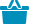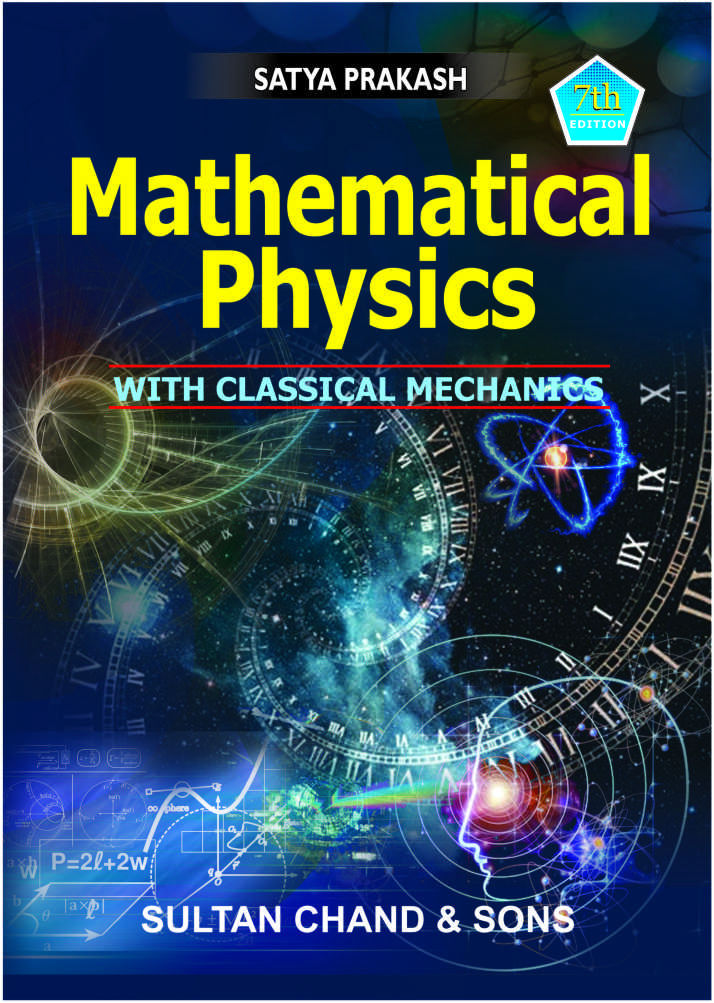Cart

# Mathematical Physics#### Quick Overview

Authored By

Publisher: Sultan Chand & Sons

Publishing Year: 2021

Size (mm): 0.00 x 0.00

ISBN: 81-8054-928-1

Page nos.: 700

MRP: 845.00

Subject: Mathematical Sciences

₹845.00

The sixth revised and enlarged edition of the book “Mathematical Physics”, To make the book more useful for Post-graduate and Engineering Students, the following changes have been made:

• Chapter 1, Vectors (with Applications) Pp. 1–132, contains 16 latest problems from examinations Question Papers.
• Chapter 2, Matrices, Pp. 133–230, contains three latest examination problems with solutions.
• Chapter 3, Tensors, Pp. 231–315, contains three latest examination problems with solutions.
• Chapter 4, The Beta, Gamma and Error Functions, Pp. 316–347, contains two latest examination problems with solutions.
• Chapter 5, Infinite Series, Pp. 348–351 –  O, contains one latest examination problem with solution.
• A large number of various University Examination Papers are added at the end of the book.
• An index is also provided at the end of the book for the ease of the students and teachers.

• Vectors (with applications)
• Matrices
• Tensors
• Beta-gamma and Error Functions
• Infinite series
• Complex Variables
• Differential Equations and Special Functions
• Fourier Series and Integrals
• Partial Differential Equations in Physics
• Fourier’s and Laplace’s Integral Transforms
• Dirac Delta Function & Green’s Function
• Probability, Statistics and Theory or Errors
• Group Theory
• Numerical Analysis
• Classical Mechanics
• Special Theory of Relativity
• Quantum Mechanics

ISBN13: 978-81-8054-928-1

Weight: 2000.00

Edition: 7th Revised and Enlarged Edition 2021

Language: English

Title Code: 587

#### Author

Authored By : Prakash Satya